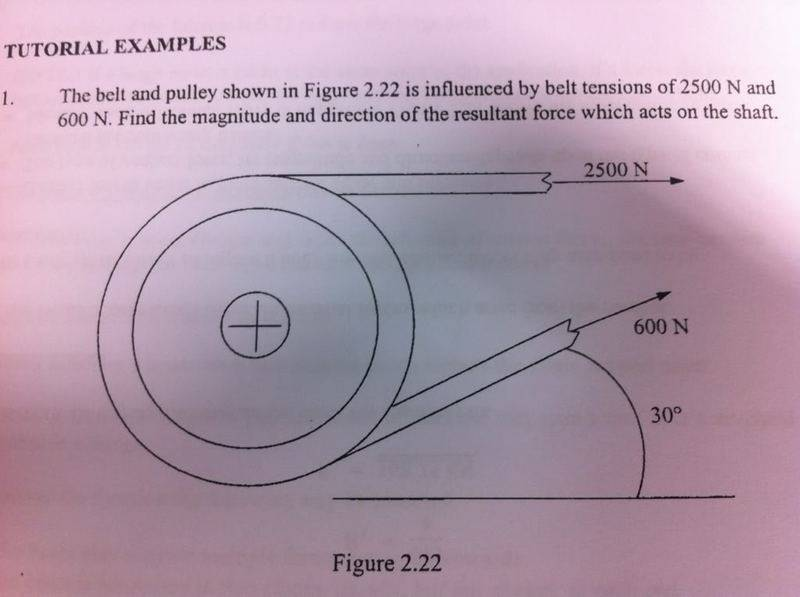# Belt & Pulley Question

• Idrees27
In summary, the conversation is about a question regarding a simple belt and pulley. The individual is trying to find the magnitude using an equation they found online, but is unsure if it is correct. They are also looking for help on how to approach the question.

#### Idrees27

Hi guys,

I am working on a simple belt and pulley question, as pictured below.To find the magnitude, I used the following equation:

(g(M-m))/(M+m)

So,

9.81(2500-600)/(2500+600) = 6.01 - Answer = 6kN

Is this correct, or am I doing it totally wrong? I have searched for the same question but didn't find any like this with a single pulley, all other examples seem to show 2 pulleys.

Any help would be greatly appreciated.

Idrees27 said:
To find the magnitude, I used the following equation:

(g(M-m))/(M+m)
I don't understand that expression. Where did you get it? (Are m and M masses?)

In any case, why not just find the net force on the pulley due to the belt tension? Add up those tension forces vectorially.

Doc Al said:
I don't understand that expression. Where did you get it? (Are m and M masses?)

In any case, why not just find the net force on the pulley due to the belt tension? Add up those tension forces vectorially.
Yes, they are masses. That's a formula I found via Google.

How would you attempt the whole question?

Idrees27 said:
Yes, they are masses. That's a formula I found via Google.
Masses of what? How is that relevant?
How would you attempt the whole question?
Do as I suggested in my last post.